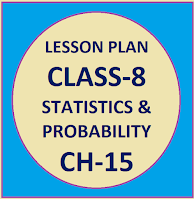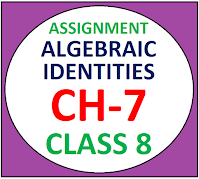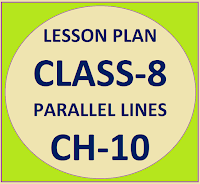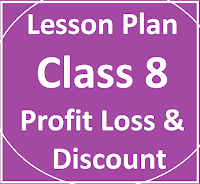## Posts

Showing posts from August, 2023

### Resource Centre Mathematics

Resource Centre Mathematics Mathematics worksheet, mathematics basic points and formulas, mathematics lesson plan, mathematics multiple choice questions Workplace Dashboard CBSE Syllabus For Session 2023-24 For  :   Classes IX & X    |   Classes XI & XII Watch Videos on Maths Solutions CLASS IX MATHEMATICS FORMULAS &  BASIC CONCEPTS

### Lesson Plan Math Class 8 | Statistics and ProbabilityE- LESSON PLAN   SUBJECT MATHEMATICS    CLASS- 8 Lesson Plan for CBSE mathematics class 8 statistics and probability, Step by step teaching strategy for mathematics teachers. Perfect lesson plan which makes the teaching learning process perfect E-LESSON PLAN MATHEMATICS CLASS-VIII CHAPTER - 15 STATISTICS & PROBABILITY NAME OF THE TEACHER DINESH KUMAR CLASS VIII CHAPTER 15 SUBJECT MATHEMATICS TOPIC STATISTICS & PROBABILITY  DURATION : 7 Class Meetings PREREQUISITE KNOWLEDGE FOR THIS LESSON PLAN Students should have a strong grasp of basic arithmetic operations such as addition, subtraction, multiplication, and division. Students should be familiar with reading and interpreting different types of graphs, such as bar graphs, line graphs, and pie charts. This understanding will help them make sense of the visual representations used in statistics. A solid understanding of fractions and decimals is important for calculating probabilities. MATERIALS REQUIRED Whiteboard and markers,

### Math Assignment Class VIII | Algebraic Identities Ch-7Mathematics Assignment  Class VIII  Algebraic Identities Download or Print, free  assignment with answer key  for   Class  8  on ALGEBRAIC IDENTITIES .   Important and extra questions that cover all topics of ALGEBRAIC IDENTITIES and is useful and helpful for the students.

### Lesson Plan Math Class 8 | Parallel Lines Ch-10E- LESSON PLAN   SUBJECT MATHEMATICS    CLASS- 8 Lesson Plan for CBSE mathematics class 8 Parallel Lines, Step by step teaching strategy for mathematics teachers. Perfect lesson plan which makes the teaching learning process perfect E-LESSON PLAN MATHEMATICS CLASS-VIII CHAPTER - 10 PARALLEL LINES NAME OF THE TEACHER DINESH KUMAR CLASS VIII CHAPTER 10 SUBJECT MATHEMATICS TOPIC PARALLEL LINES  DURATION : 10 Class Meetings SUB-TOPICS TO BE ADRESSED Define parallel lines and identify them in various geometrical figures. Identify properties of parallel lines and their corresponding angles. Solve problems involving parallel lines and transversals. Divide the given line segment into different equal parts.

### Math Assignment Class VIII | Profit, Loss and Discount CH-5Math Assignment  Class VIII | Profit, Loss & Discount

### Lesson Plan Math Class 8 | Profit, loss & Discount CH-5E- LESSON PLAN   SUBJECT MATHEMATICS    CLASS- 8 Lesson Plan for CBSE mathematics class 8 Profit, Loss and Discount, Step by step teaching strategy for mathematics teachers. Perfect lesson plan which makes the teaching learning process perfect E-LESSON PLAN MATHEMATICS CLASS-VIII CHAPTER-3 PROFIT, LOSS & DISCOUNT NAME OF THE TEACHER DINESH KUMAR CLASS VIII CHAPTER 05 SUBJECT MATHEMATICS TOPIC PROFIT, LOSS & DISCOUNT  DURATION : 10 Class Meetings PRE- REQUISITE KNOWLEDGE: Students should have a strong foundation in basic arithmetic operations, including addition, subtraction, multiplication, and division. They should be comfortable working with whole numbers, decimals, and fractions. Students should understand the concept of percentages and how to convert percentages to decimals and fractions. This will be crucial for calculating discounts and determining profit or loss percentages. A basic understanding of ratios will be helpful as some problems may involve using ratios to ca